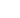#Management Accounting: Decision and Control Test One Q1 of 15

The following information has been calculated for the production of 1 unit of Gamma
• Each unit will require 0.35 kilograms of material at a total cost of £14.00
• Each unit will require 45 minutes of labour at a cost of £12.00 per hour
• Fixed overheads total £63,000 and the estimated output will be 12,000 units of Gamma
• Fixed overheads are absorbed on a labour hour basis

Complete the standard cost card below. Show all figures to 2 decimal places.

 1 unit of Gamma Quantity Cost per unit £ Total cost £ Material kgLabour hoursFixed costs hoursTotals